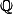# Martin Orr's Blog

## Endomorphisms of simple abelian varieties

Posted by Martin Orr on Thursday, 03 January 2013 at 15:51

Today I will discuss the classification of endomorphism algebras of simple abelian varieties. The endomorphism algebra of a non-simple abelian variety can easily be computed from the endomorphism algebras of its simple factors. For a simple abelian variety, its endomorphism algebra is a division algebra of finite dimension over ``. (A division algebra is a not-necessarily-commutative algebra in which every non-zero element is invertible.) As discussed last time, the endomorphism algebra also has a positive involution, the Rosati involution. There may be many Rosati involutions, coming from different polarisations of the abelian variety, but all we care about today is the existence of a positive involution. Division algebras with positive involutions were classified by Albert in the 1930s.